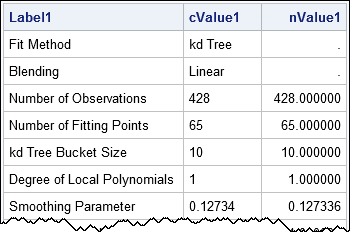Have you ever seen the "Fit Summary" table from PROC LOESS, as shown to the right? Or maybe you've seen the "Model Information" table that is displayed by some SAS analytical procedures? These tables provide brief interesting facts about a statistical procedure, hence they are called factoids.

In SAS, a "factoid" has a technical meaning:

• A factoid is an ODS table that can display numerical and character values in the same column.
• A factoid displays row headers in a column. In most ODS styles, the row headers are displayed in the first column by using a special style, such as a light-blue background color in the HTMLBlue style.
• A factoid does not display column headers because the columns display disparate and potentially unrelated information.

I want to emphasize the first item in the list. Since variables in a SAS data set must be either character or numeric, you might wonder how to access the data that underlies a factoid. You can use the ODS OUTPUT statement to look at the data object behind any SAS table, as shown below:

```proc loess data=sashelp.cars plots=none; model mpg_city = weight; ods output FitSummary=Fit(drop=SmoothingParameter); run;   proc print data=Fit noobs; run;```The PROC PRINT output shows how the factoid manages to display characters and numbers in the same column. The underlying data object contains three columns. The LABEL1 column contains the row headers, which identify each row. The CVALUE1 column is the column that is displayed in the factoid. It is a character column that contains character strings and formatted copies of the numbers in the NVALUE1 column. The NVALUE1 column contains the raw numeric value of every number in the table. Missing values represent rows for which the table displays a character value.

All factoids use the same naming scheme and display the LABEL1 and CVALUE1 columns. The form of the data is important when you want to use the numbers from a factoid in a SAS program. Do not use the CVALUE1 character variable to get numbers! Those values are formatted and possibly truncated, as you can see by looking at the "Smoothing Parameter" row. Instead, read the numbers from the NVALUE1 variable, which stores the full double-precision number.

For example, if you want to use the AICC statistic (the last row) in a program, read it from the NVALUE1 column, as follows:

```data _NULL_; set Fit(where=( Label1="AICC" )); /* get row with AICC value */ call symputx("aicc", NValue1); /* read value of NValue1 variable into a macro */ run; %put &=aicc; /* display value in log */```
`AICC=3.196483775`

Some procedures produce factoids that display multiple columns. For example, PROC CONTENTS creates the "Attributes" table, which is a factoid that displays four columns. The "Attributes table displays two columns of labels and two columns of values. When you use the ODS OUTPUT statement to create a data set, the variables for the first two columns are LABEL1, CVALUE1, and NVALUE1. The variables for the second two columns are LABEL2, CVALUE2, and NVALUE2.

Be aware that the values in the LABEL1 (and LABEL2) columns depend on the LOCALE= option for your SAS session. This means that some values in the LABEL1 column might be translated into French, German, Korean, and so forth. When you use a WHERE clause to extract a value, be aware that the WHERE clause might be invalid in other locales. If you suspect that your program will be run under multiple locales, use the _N_ automatic variable, such as if _N_=14 then call symputx("aicc", NValue1);. Compared with the WHERE clause, using the _N_ variable is less readable but more portable.

Now that you know what a factoid is, you will undoubtedly notice them everywhere in your SAS output. Remember that if you need to obtain numerical values from a factoid, use the ODS OUTPUT statement to create a data set. The NVALUE1 variable contains the full double-precision numbers in the factoid. The CVALUE1 variable contains character values and formatted versions of the numbers.

Share